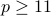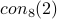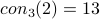# Consecutive nth powers

Definitionis the least prime such that for allthere will bea run ofconsecutiveth powers. End of Definition

We abbreviate the phrase run ofconsecutiveth powers by RkCL.

The following is in a paper by Lehmer and Lehmer (paper below) however, they seem to treat it as folklore.

Theorem.

Proof

Note that there is no R2C2 mod 5. Note that there is a R2C2 mod 7: 1,2 since.

Let. All arithmetic is mod. If 2 is a square then there is a pair, namely. If 5 is a square then there is a pair, namely. If neither is a square then the productis a square and hence

End of Proof

Note that this proof is elementary. More formally we have a bound on where the R2C2 will be: it will always start with a number that is. We call such proofs LL to honor Lehmer and Lehmer. (Using the term elementary is not good since this can mean other things.)

Many of the papers listed below show that this kind of proof is not possible for some of thevalues.

# Papers about residues

H. Davenport. On the distribution of quadratic residues mod p. Journal of the London Mathematical Society, 6:49–54, 1932. Forthe number of RkC2 is.

H. Davenport. On the distribution ofth power residues mod p. Journal of the London Mathematical Society, 7:117–121, 1932. HERE The last theorem of this paper is that ifthen there are 3 consecutiveth roots mod.

H. Davenport. On the distribution of quadratic residues mod p. Journal of the London Mathematical Society, 8:46–52, 1933. POINTER

D. Burgess. The distribution of the quadratic residues and non-residues. Mathematika, pages 106–112, 1957. HERE this paper shows that the max number of consecutive quadraic residues or non-residues modis.

D. H. Lehmer and E. Lehmer. On runs of residues. Proceedings of the American Mathematical Society, 13:102–106, 1962. This paper shows that there is no LL proof of a bound on. HERE

D. H. Lehmer, E. Lehmer, W. Mills, and J.L.Selfridge. Machine proof of a theorem on cubic residues. Mathematics of Computation, 16:407–415, 1962. This paper gives an LL proof that. HERE

R. G. Bierstedy and W. H. Mills. On the bound for a pair of consecutive quartic residues of a prime. Proceedings of the American Mathematical Society, 14:628–632, 1963. This paper gives an LL proof that. HERE

D. H. Lehmer and E. Lehmer. On runs of residues. Proceedings of the American Mathematical Society, 13:102–106, 1962. D. H. Lehmer, E. Lehmer, and W. Mills. Pairs of consecutive power residues. Canadian Journal of Mathematics, 15:172–177, 1963. This paper gives an LL proof thatand. HERE

J. Brillhart, D. H. Lehmer, and E. Lehmer. Bounds for pairs of consecutive seventh and higher powers. Mathematics of Computation, 18:397–407, 1964. This paper shows that if there is an LL proof for, (,,) then the numbers involved will be quite large (see the paper itself to make this formal). HERE

R. Graham. On quadruples of consecutive kth power residues. Proceedings of the American Mathematical Society, 15:196–197, 1964. 1964. This paper show that, for all, there is NO LL proof for. HERE

M. Dunton. Bounds for pairs of cubic residues. Proceedings of the American Mathematical Society, 16:330–332, 1965. HERE This paper gives an elementary proof that.

J. R. Rabung and J. H. Jordan. Consecutive power residues or nonresidues. Mathematics of Computation, 24:737–740, 1970. url{http:www.jstor.orgstable2004850} or url{http:www.cs.umd.edu gasarchres/}. 1970. This paper looks at the problem of finding a sequence ofth powers OR non-th powers. HERE

J. R. Rabung. On applications of van der {W}aerden's theorem. Mathematical Magazine, 48:142–148, 1975. HERE This paper looks at these kind of problems within the Gaussian Integers.

R. Peralta. On the distribution of quadratic residues and nonresidues modulo a prime number. Mathematics of Computation, 58:433–440, 1992. 1992. This paper shows that. It is not stated that way. HERE

# Papers about FLT

G. Libri. Me"moire sur la the"orie des nombres. Journal f{"{u}}r die reine und angewandte Mathematik, pages 54–81, 1832. HERE

P. Pepin. E'tude sur la the'orie des re'sidues cubiques. Journal de {Math'{e}matiques} Pures et {Appliqu'{e}es}, 2:313–324, 1876. HERE

P. Pepin. Sur divers tentatives de de'monstration du the'ore'me de {F}ermat. Comptes Rendus de l'Acad{'{e}}mie des Sciences Paris, HERE

A. E. Pellet. Me'morire sur la the'orie alge'brique des e'quations. Bulletin de la societe Mathematique de France, pages 61–103, 1887. HERE

L. E. Dickson. Lower limit for the number of sets of solutions of. Journal f{"{u}}r die reine und angewandte Mathematik, pages 181–189, 1909. HERE

G. Cornacchia. Sulla congruenza. Giornale di matematiche di Battaglini, pages 219–268, 1909. HERE

A. Hurwitz. U"ber die kongruenz. Journal f{"{u}}r die reine und angewandte Mathematik, pages 272–292, 1909. HERE